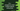# C++ program to store student details using class## Introduction:

In this `C++` tutorial, we will learn how to read the details of students and how to print them using a `Class`. We will use one `class` to hold the name and marks of each student and two member functions to read and print these details.

I am explaining the steps at the end of this article. You can go through the steps to learn how the program works.

### C++ program to record and print student details:

The following program stores the name and marks of any number of students and prints them. It reads the values as inputs from the user.

``````#include <iostream>
#include <string>
using namespace std;

// 1
class Student
{
private:
string name;
int marks;

public:
void getDetails();
void setDetails();
};

// 2
void Student::setDetails()
{
cout << "Enter the name:" << endl;
cin >> name;
cout << "Enter total marks:" << endl;
cin >> marks;
}

// 3
void Student::getDetails()
{
cout << "Name: " << name << " ,marks: " << marks << endl;
}

int main(int argc, char const *argv[])
{
// 4
int count;
cout << "Enter the total number of students: ";
cin >> count;

// 5
if (count > 0)
{
// 6
Student studentArray[count];
for (int i = 0; i < count; i++)
{
cout << "For student " << i + 1 << ":" << endl;
studentArray[i].setDetails();
}

// 7
cout << "\nYou have entered:" << endl;
for (int i = 0; i < count; i++)
{
studentArray[i].getDetails();
}
}
else
{
cout << "Please enter a valid number." << endl;
}
return 0;
}``````

#### Explanation :

The commented numbers in the above program denote the step numbers below:

1. The above example is using a class, `Student`, with two private variables and two public methods. The variable `name` and `marks` are used to store the name and marks of a student and the methods `getDetails` and `setDetails` are used to get the details and assign values to the private variables.

2. `setDetails` is a member function to assign values to the variable `name` and `marks`. If you call this function, it will ask the user to enter the name and marks. With `cin`, we are assigning these values to the variable `name` and `marks`.

3. `getDetails` member function is used to print the details. It prints the `name` and `marks` for that class object.

4. The `main` function is the starting point of the program. It asks the user to enter the total number of students. This value is assigned to the `count` variable.

5. Check if the entered value is greater than 0 or not. If not, print one message to enter a valid number.

6. If the entered value is greater than 0, create one array of `Student` to hold the names and marks of the students. By using one `for` loop, we are assigning the values to the variables of the class objects. It calls the `setDetails` function of a `Student` object to read these values.

7. It uses one more `for` loop to print the user-entered values. We are using the `getDetails` method to print the name and marks.

#### Sample output:

If you run the above program, it will print outputs as below:

##### Example 1:
``````Enter the count of students : 4
For student 1 :
Enter the name :
Alex
Enter total marks :
88
For student 2 :
Enter the name :
Bob
Enter total marks :
77
For student 3 :
Enter the name :
Charlie
Enter total marks :
66
For student 4 :
Enter the name :
Dan
Enter total marks :
59

You have entered :
Name : Alex ,marks : 88
Name : Bob ,marks : 77
Name : Charlie ,marks : 66
Name : Dan ,marks : 59``````
##### Example 2:
``````Enter the count of students : -3
We are using the `Student` class to hold only the name and marks of a student. You can extend this program to hold any other information as well. Similarly, you can add more member functions to the class.